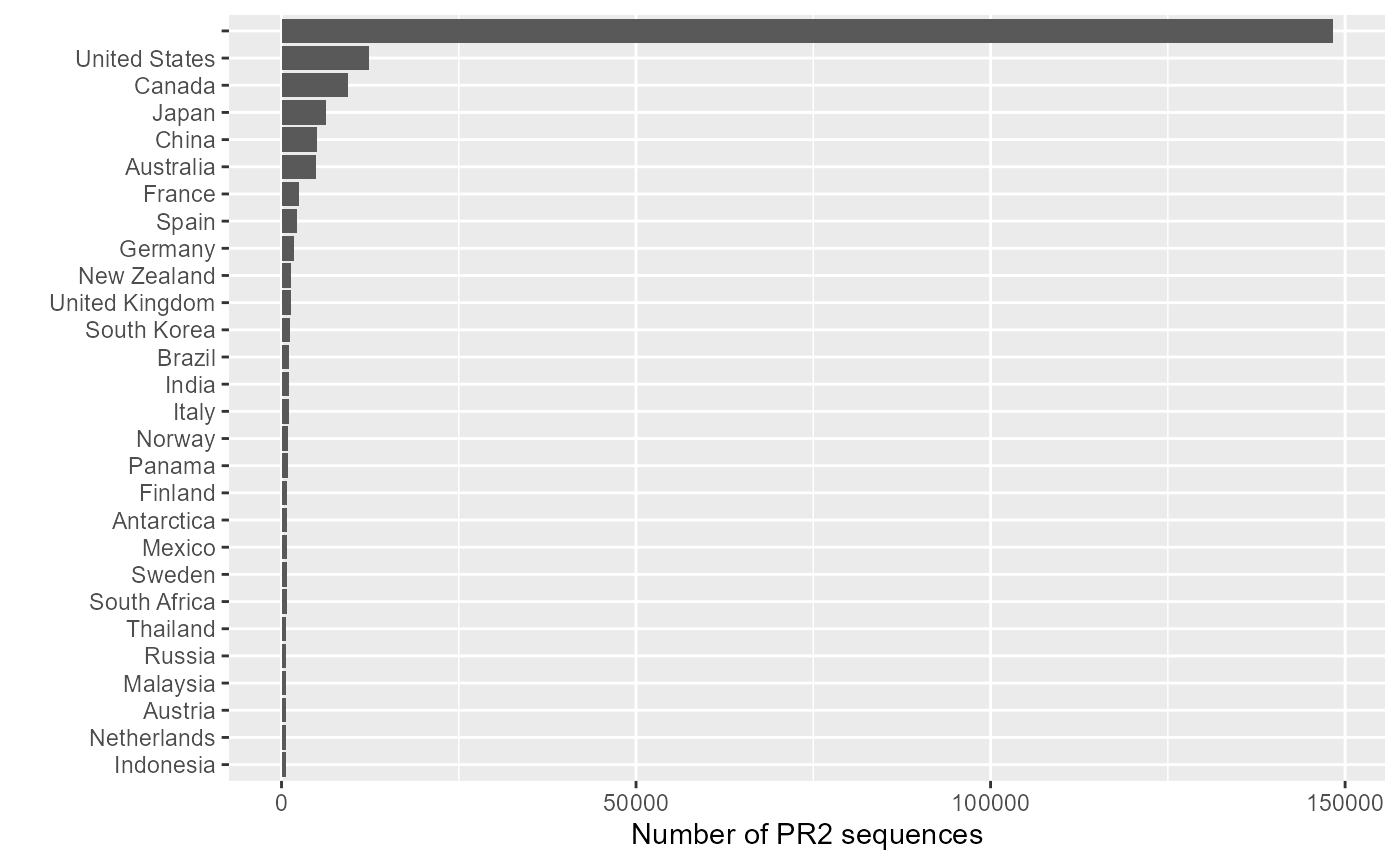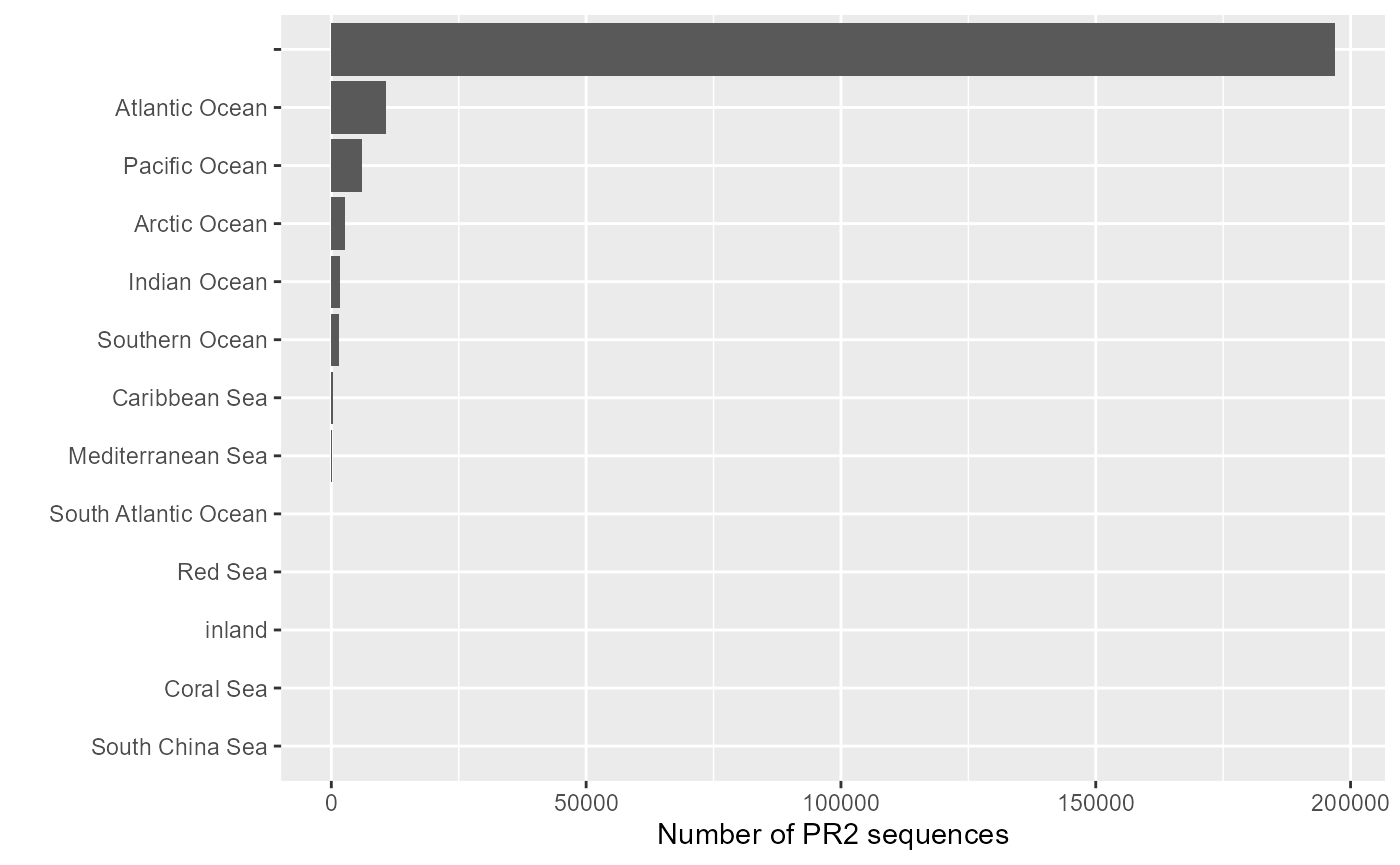library("pr2database")

pr2 <- pr2_database()

  library(dplyr)
library(ggplot2)    # For plots

## Plotting number of sequences per country and ocean

A very good tutorial by Margaret Mars Brisbin on how to combine PR2 metadata with Python to locate sequences using all the metadata information (lat, long, country and fuzzy localization): https://maggimars.github.io/eukGeoBlast/eGB.html and https://github.com/maggimars/eukGeoBlast. This code has been used to incorporate more geo-localisation information into PR2 version 4.12.0

### Number of sequences per country of origin


pr2 %>%
count(pr2_country) %>%
filter(!is.na(pr2_country) & n > 500) %>%
ggplot(aes(x = reorder(pr2_country, n), y = n)) +
geom_col() +
coord_flip() +
xlab("") +
ylab("Number of PR2 sequences")### Number of sequences per ocean of origin


oceans <- pr2 %>%
count(pr2_ocean) %>%
filter(!is.na(pr2_ocean))

ggplot(oceans, aes(x = reorder(pr2_ocean, n), y = n)) +
geom_col() +
coord_flip() +
xlab("")  + ylab("Number of PR2 sequences")